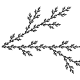You are currently browsing the tag archive for the ‘Duistermaat-Heckmann measure’ tag.

Let${A, B}$ be${n \times n}$ Hermitian matrices, with eigenvalues${\lambda_1(A) \leq \ldots \leq \lambda_n(A)}$ and${\lambda_1(B) \leq \ldots\leq \lambda_n(B)}$. The Harish-ChandraItzykson-Zuber integral formula exactly computes the integral$\displaystyle \int_{U(n)} \exp( t \hbox{tr}( A U B U^* ) )\ dU$

where${U}$ is integrated over the Haar probability measure of the unitary group${U(n)}$ and${t}$ is a non-zero complex parameter, as the expression$\displaystyle c_n \frac{ \det( \exp( t \lambda_i(A) \lambda_j(B) ) )_{1 \leq i,j \leq n} }{t^{(n^2-n)/2} \Delta(\lambda(A)) \Delta(\lambda(B))}$

when the eigenvalues of${A,B}$ are simple, where${\Delta}$ denotes the Vandermonde determinant$\displaystyle \Delta(\lambda(A)) := \prod_{1 \leq i

and${c_n}$ is the constant$\displaystyle c_n := \prod_{i=1}^{n-1} i!.$

There are at least two standard ways to prove this formula in the literature. One way is by applying the Duistermaat-Heckman theorem to the pushforward of Liouville measure on the coadjoint orbit${{\mathcal O}_B := \{ UBU^*: U \in U(n) \}}$ (or more precisely, a rotation of such an orbit by${i}$) under the moment map${M \mapsto \hbox{diag}(M)}$, and then using a stationary phase expansion. Another way, which I only learned about recently, is to use the formulae for evolution of eigenvalues under Dyson Brownian motion (as well as the closely related formulae for the GUE ensemble), which were derived in this previous blog post. Both of these approaches can be found in several places in the literature (the former being observed in the original paper of Duistermaat and Heckman, and the latter observed in the paper of Itzykson and Zuber as well as in this later paper of Johansson), but I thought I would record both of these here for my own benefit.

The Harish-Chandra-Itzykson-Zuber formula can be extended to other compact Lie groups than${U(n)}$. At first glance, this might suggest that these formulae could be of use in the study of the GOE ensemble, but unfortunately the Lie algebra associated to${O(n)}$ corresponds to real anti-symmetric matrices rather than real symmetric matrices. This also occurs in the${U(n)}$ case, but there one can simply multiply by${i}$ to rotate a complex skew-Hermitian matrix into a complex Hermitian matrix. This is consistent, though, with the fact that the (somewhat rarely studied) anti-symmetric GOE ensemble has cleaner formulae (in particular, having a determinantal structure similar to GUE) than the (much more commonly studied) symmetric GOE ensemble.Usman Nizami on AppletsAnonymous on 247B, Notes 3: pseudodifferent…Xiaoyan Su on 247B, Notes 3: pseudodifferent…Rex on 247B, Notes 4: almost everywhe…Rex on 247B, Notes 4: almost everywhe…Terence Tao on 254A, Notes 1: Local well-pose…Terence Tao on The completeness and compactne…Terence Tao on 247B, Notes 3: pseudodifferent…Terence Tao on 247B, Notes 4: almost everywhe…John Mangual on 247B, Notes 4: almost everywhe…Liam Shakib Llamazar… on 254A, Notes 1: Local well-pose…Alan Chang on 247B, Notes 4: almost everywhe…Anonymous on 247B, Notes 1: Restriction…Anonymous on 247B, Notes 4: almost everywhe…Anonymous on 247B, Notes 1: Restriction…